• 文章目录初等变换初等矩阵等价标准形参考 初等变换 定义\large\color{magenta}{\boxed{\color{brown}{定义} }}定义​ 下面的三种变换叫做 λ\lambdaλ -矩阵初等变换: (1) 矩阵的两行（列）互换位置; (换行） (2) ...
文章目录初等变换初等矩阵相抵 (等价)相抵标准形参考

80相似标准形01——lambda矩阵
81相似标准形02——初等变换、初等矩阵、相抵 (等价)、相抵标准形
82相似标准形03——不变因子、行列式因子、相抵标准形的唯一性、用求行列式因子法求标准形
83相似标准形04——相似与λ-矩阵的相抵
84相似标准形05——有理标准形的不变因子、矩阵的有理标准形
85相似标准形06——初等因子、初等因子与不变因子的求法
86相似标准形07——若尔当(Jordan)标准形
87相似标准形08——Jordan标准形
88相似标准形09——JJordan-Chevalley分解、幂零矩阵与幂零变换、幂零矩阵的判别、中国剩余定理、可换线性变换的性质
89相似标准形10——J循环不变子空间

初等变换
$\large\color{magenta}{\boxed{\color{brown}{定义1} }}$ 下面的三种变换叫做 $\lambda$ -矩阵的初等变换:
(1) 矩阵的两行（列）互换位置; (换行）
(2) 矩阵的某一行（列）乘以非零的常数 $c ;$ (倍行）
(3) 矩阵有某一行 (列）加另一行 (列）的 $\varphi(\lambda)$ 倍， $\varphi(\lambda)$ 是一个多项式。 $($ 倍法化零)
初等矩阵
$\large\color{magenta}{\boxed{\color{brown}{定义2} }}$ 和数字矩阵的初等变换一样，可以引进初等矩阵。

互换矩阵：  用 $P(i, j)$ 表示由单位矩阵经过第 $i$ 行第 $j$ 行互换位置所得的初等矩阵，倍法矩阵 ：用$P(i(c))$ 表示用非零常数 $c$ 乘单位矩阵第 $i$ 行所得的初等矩阵。消法矩阵 ：将单位矩阵的第 $j$行乘一个多项式$\varphi(\lambda)$ 加到第 $i$ 行上，记为$P(i, j(\varphi(\lambda)))$   得同数字矩阵的证明一样, 有
$\large\color{magenta}{\boxed{\color{brown}{命题1} }}$同数字矩阵一样,对一个 $s \times n$ 的 $\lambda$ -矩阵 $A(\lambda)$ 作一次初等行变换就相当于在$A(\lambda)$ 的左边乘上相应 $s \times s$ 的初等矩阵; 对 $A(\lambda)$ 作一次初等列变换就相当于 $A(\lambda)$在的右边乘上相应的 $n \times n$ 的初等矩阵。
同数字矩阵一样可以证明:  对  $\lambda$ -矩阵作初等变换不改变 $\lambda$ -矩阵的秩.
$\large\color{magenta}{\boxed{\color{brown}{性质1} }}$  初等  $\lambda$ -矩阵是可逆的, 且它们的逆也是初等  $\lambda$ -矩阵
$P(i, j)^{-1}=P(i, j), \\P(i(c))^{-1}=P\left(i\left(c^{-1}\right)\right), \\P(i, j(\varphi))^{-1}=P(i, j(-\varphi)) \text { 。 }$
由此得出初等变换具有可逆性：设 $\lambda$ -矩阵 $A(\lambda)$ 用初等变换变成 $B(\lambda)$, 这相当于对 $A(\lambda)$ 左乘或右乘一个初等矩阵.再用此初等矩阵的逆矩阵来乘 $B(\lambda)$ 就变回 $A(\lambda)$, 而这逆矩阵仍是初等矩阵，因而由 $B(\lambda)$ 可用初等变换变回 $A(\lambda)$ 。
相抵 (等价)
$\large\color{magenta}{\boxed{\color{brown}{定义3} }}$  $\lambda-$ 矩阵 $A(\lambda)$ 经过一系列初等变换将$A(\lambda)$ 化为 $B(\lambda)$ 记为 $A(\lambda) \rightarrow B(\lambda)$ ，称 $A(\lambda)$  与 $B(\lambda)$ $\large\color{red}{\boxed{\color{green}{ 相抵 }}}$ (等价)，
$\Large\color{violet}{注：}$ 相抵 (等价)是 $\lambda$ -矩阵之间的一种关系，
$\large\color{magenta}{\boxed{\color{brown}{性质3} }}$ (1) 反身性：每一个 $\lambda$ -矩阵与它自身相抵。
(2) 对称性: 若 $A(\lambda)$ 与 $B(\lambda)$ 相抵，则 $B(\lambda)$ 与 $A(\lambda)$ 相抵。
(3) 传递性: 若 $A(\lambda)$ 与 $B(\lambda)$ 相抵， $B(\lambda)$ 与 $C(\lambda)$ 相抵，则 $A(\lambda)$ 与 $C(\lambda)$ 相抵。
$\Large\color{violet}{例 1}$  证明 $\left(\begin{array}{ll}d_{1}(\lambda) & \\ & d_{2}(\lambda)\end{array}\right) 与\left(\begin{array}{ll}d_{2}(\lambda) & \\ & d_{1}(\lambda)\end{array}\right)$ 相抵
$\left(\begin{array}{ll}d_{1}(\lambda) & \\ & d_{2}(\lambda)\end{array}\right) \rightarrow\left(\begin{array}{cc}0 & d_{2}(\lambda) \\ d_{1}(\lambda) & 0\end{array}\right)\\ \rightarrow\left(\begin{array}{ll}d_{2}(\lambda) & \\ & d_{1}(\lambda)\end{array}\right)$
$\Large\color{violet}{注：}$ 设 $A(\lambda)=\operatorname{diag}\left(d_{1}(\lambda), d_{2}(\lambda), \cdots, d_{n}(\lambda)\right)$$\quad B(\lambda)=\operatorname{diag}\left(d_{i}(\lambda), d_{i_{2}}(\lambda), \cdots, d_{i_{n}}(\lambda)\right)$,
其中 $d_{i_{1}}(\lambda), d_{i_{2}}(\lambda), \cdots, d_{i_{n}}(\lambda)$ 是 $d_{1}(\lambda), d_{2}(\lambda), \cdots, d_{n}(\lambda)$的一个置换, 则同理可证 $A(\lambda) \simeq \boldsymbol{B}(\lambda) .$
$\large\color{magenta}{\boxed{\color{brown}{相抵的充要条件} }}$
应用初等变换与初等矩阵的关系即得，矩阵 $A(\lambda)$ 与 $B(\lambda)$ 相抵的充要条件为有一系列初等矩阵 $P_{1}, P_{2}, \cdots, P_{l}, Q_{1}, Q_{2}, \cdots, Q_{t}$, 使
$A(\lambda)=P_{1} P_{2} \cdots P_{l} B(\lambda) Q_{1} Q_{2} \cdots Q_{t}$
相抵标准形
这一节主要是证明任意一个 $\lambda$ - 矩阵可以经过初等变换化为某种对角形。为此，首先给出下面的引理.
$\large\color{magenta}{\boxed{\color{brown}{引理1} }}$   设 $A(\lambda)=\left(a_{i j}(\lambda)\right)_{m \times n}$ 为一非零$\lambda$-矩阵,若某 $a_{i j}(\lambda)$ 不是 $a_{11}(\lambda)$ 的倍式, 则 $A(\lambda)$必相抵于矩阵 $\boldsymbol{B}(\lambda)=\left(\boldsymbol{b}_{i j}(\lambda)\right)_{m \times n}$, 其中 $b_{11}(\lambda) \neq \mathbf{0}$,且 $b_{11}(\lambda)$ 整除 $\boldsymbol{B}(\lambda)$ 中的任意元 $\boldsymbol{b}_{i j}(\lambda)$,且 $\quad 0 \leq \operatorname{deg}\left(b_{11}(\lambda)\right)<\operatorname{deg}\left(a_{11}(\lambda)\right) .$
$\lambda-$ 矩阵 $A(\lambda)$ 的左上角元素 $a_{11}(\lambda) \neq 0$ ，并且 $A(\lambda)$ 中至少有一个元素不能被它除尽 (不全是它的公倍数)，那么一定可以找到一个与 $A(\lambda)$ 相抵的矩阵 $B(\lambda)$, 它的左上角元素也不为零，但是次数比 $a_{11}(\lambda)$ 的次数低。
【证明】： 根据 $A(\lambda)$ 中不能被 $a_{11}(\lambda)$ 除尽的元素所在的位置，分三种情况来讨论:

若 $A(\lambda)$ 的第一列中有一个元素 $a_{i 1}(\lambda)$ 不能被 $a_{11}(\lambda)$ 除尽，则有
$a_{i 1}(\lambda)=a_{11}(\lambda) q(\lambda)+r(\lambda)$
其中余式 $r(\lambda) \neq 0$, 且次数比 $a_{11}(\lambda)$ 的次数低.

对 $A(\lambda)$ 作初等行变换. $\quad$ 把 $A(\lambda)$ 的第 $i$ 行减去第 1 行的 $q(\lambda)$ 倍，得
$A(\lambda)=\left(\begin{array}{cc} a_{11}(\lambda) & \cdots \\ \vdots & \vdots \\ a_{i 1}(\lambda) & \cdots \\ \vdots & \vdots \end{array}\right) \longrightarrow\left(\begin{array}{cc} a_{11}(\lambda) & \cdots \\ \vdots & \vdots \\ r(\lambda) & \cdots \\ \vdots & \vdots \end{array}\right)$
再将此矩阵的第 1 行与第 $i$ 行互换，得:
$A(\lambda) \longrightarrow\left(\begin{array}{cc} r(\lambda) & \cdots \\ \vdots & \vdots \\ a_{11}(\lambda) & \cdots \\ \vdots & \vdots \end{array}\right)=B(\lambda)$
$B(\lambda)$ 左上角元素 $r(\lambda)$ 符合引理的要求，故 $B(\lambda)$ 即为所求。

在 $A(\lambda)$ 的第一行中有一个元素 $a_{1 i}(\lambda)$ 不能被 $a_{11}(\lambda)$ 除尽，这种情况的证明与 1$)$ 类似，但是
对 $A(\lambda)$ 进行的是初等列变换.

$A(\lambda)$ 的第一行与第一列中的元素都可以被$a_{11}(\lambda)$ 除尽，但 $A(\lambda)$ 中有另一个元素 $a_{i j}(\lambda)(i>1,$$j>1$ ) 不能被 $a_{11}(\lambda)$ 除尽。 设
$a_{i 1}(\lambda)=a_{11}(\lambda) \varphi(\lambda)$
对 $A(\lambda)$ 作下述初等行变换：
$\begin{array}{c} A(\lambda)=\left(\begin{array}{cccc} a_{11}(\lambda) & \cdots & a_{1 j}(\lambda) & \cdots \\ \vdots & & \vdots & \vdots \\ a_{i 1}(\lambda) & \cdots & a_{i j}(\lambda) & \cdots \\ \vdots & & \vdots & \vdots \end{array}\right) \\ 第一行的 -\varphi(\lambda)加到i行 \longrightarrow\left(\begin{array}{cccc} a_{11}(\lambda) & \cdots & a_{1 j}(\lambda) & & \cdots \\ \vdots & & & \vdots & & \vdots \\ 0 & \cdots & a_{i j}(\lambda)-a_{1 j}(\lambda) \varphi(\lambda) & \cdots \\ \vdots & & & \vdots & & \vdots \end{array}\right) \end{array}$

$\begin{array}{ll} i行加到 1行 \longrightarrow\left(\begin{array}{cccc} a_{11}(\lambda) & \cdots & a_{i j}(\lambda)+(1-\varphi(\lambda)) a_{1 j}(\lambda) & \cdots \\ \vdots & & & \vdots & \vdots \\ 0 & \cdots & a_{i j}(\lambda)-a_{1 j}(\lambda) \varphi(\lambda) & \cdots \\ \vdots & & \vdots & \vdots \end{array}\right) \\ =A_{1}(\lambda) . & & & & \end{array}$
矩阵 $A_{1}(\lambda)$ 的第一行中，有一个元素
$a_{i j}(\lambda)+(1-\varphi(\lambda)) a_{1 j}(\lambda)$
不能被左上角元素 $a_{11}(\lambda)$ 除尽，这就化为已经证明了的情况 2).
$\large\color{magenta}{\boxed{\color{brown}{推论1} }}$ $A(\lambda) \neq O \Rightarrow$ 相抵于 $C(\lambda)=\left(\begin{array}{cc}c_{11}(\lambda) & O \\ O & C_{1}(\lambda)\end{array}\right)$ 使得$c_{11}(\lambda) \neq 0$, 且 $C_{1}(\lambda)$ 中所有元都是 $c_{11}(\lambda)$ 的倍式.
证明: $\Rightarrow$ 存在相抵矩阵 $B(\lambda)=\left(b_{i j}(\lambda)\right)$, 使得 $b_{11}(\lambda)$ 整除 $B(\lambda)$ 中所有元
$\left.\begin{array}{l}\text { 将 } B(\lambda) \text { 第 } 1 \text { 行的适当倍数加到下面的 行 } \\ \text { 将 } B(\lambda) \text { 第 } 1 \text { 列的适当倍数加到右面的列 }\end{array}\right\} \Rightarrow$ 得所需之 $C(\lambda)$
$c_{11}(\lambda)=?$ 三种初等变换不会改变 $\lambda$ -矩阵所有元的最大公因式
$c_{11}(\lambda)$ 与 $C(\lambda)$ 所有元的最大公因式相差非零常数倍
$\Rightarrow c_{11}(\lambda)$ 与 $A(\lambda)$ 所有元的最大公因式相差非零常数倍！
$A(\lambda) \stackrel{\text { 相抵于 }}{\longrightarrow}\left(\begin{array}{cc}d_{1}(\lambda) & o \\ o & D_{1}(\lambda)\end{array}\right) \begin{array}{c}\text { 其中 } d_{1}(\lambda) \text { 整除 } D_{1}(\lambda) \text { 所有元, 且 } d_{1}(\lambda) \text { 与 } A(\lambda) \\ \text { 所有元的最大公因式相差非零常数 倍 }\end{array}$
$D_{1}(\lambda) \stackrel{\text { 相抵干 }}{\longrightarrow}\left(\begin{array}{cc}d_{2}(\lambda) & O \\ O & D_{2}(\lambda)\end{array}\right)$ 其中 $d_{2}(\lambda)$ 整除 $D_{2}(\lambda)$ 所有元, 且 $d_{2}(\lambda)$ 与 $D_{1}(\lambda)$ 所有元的最大公因式相差常数倍
特别地, $d_{1}(\lambda) \mid d_{2}(\lambda) \quad$…重复如上过程 $\ldots \ldots$
$\stackrel{\text { 相执于 }}{\longrightarrow} D(\lambda)=\left(\begin{array}{llll} d_{1}(\lambda) & & & & \\ & d_{2}(\lambda) & & & o \\ & & \ddots & & \\ & & & d_{r}(\lambda) & \\ & & o & & o \end{array}\right), \text { 其中 } d_{i}(\lambda) \mid d_{i+1}(\lambda)(1 \leq i \leq r-1)$
$\large\color{magenta}{\boxed{\color{brown}{定理2} }}$   任意一个非零的 $s \times n$ 的 $\lambda-$ 矩阵 $A(\lambda)$ 都相抵于下列形式的矩阵 ,$r(A(\lambda))=r$
$\left(\begin{array}{llllll} d_{1}(\lambda) & & & & & & \\ & d_{2}(\lambda) & & & & \\ & & \ddots & & & & \\ & & & d_{r}(\lambda) & & & \\ & & & & 0 & & \\ & & & & & \ddots & \\ & & & & & & 0 \end{array}\right),$
其中 $d_{i}(\lambda)(i=1,2, \cdots, r)$ 是首项系数为 1 的多项式，且
$d_{i}(\lambda) \mid d_{i+1}(\lambda) \quad(i=1,2, \cdots, r-1) .$
这个矩阵称为 $A(\lambda)$ 的相抵标准形
注：我把这种方法称为“降次剥皮法”
$\large\color{magenta}{\boxed{\color{brown}{推论2} }}$任 - $\boldsymbol{n}$ 阶可逆 $\lambda$-矩阵都可表示为有限个初等$\lambda$-矩阵之积
设 $A(\lambda)$ 是 $n$ 阶可逆$\lambda$-矩阵,则存在 $P_{1}(\lambda), \cdots, P_{s}(\lambda), \boldsymbol{Q}_{1}(\lambda), \cdots, \boldsymbol{Q}_{t}(\lambda)$, 使得
$\boldsymbol{P}_{s}(\lambda) \cdots \boldsymbol{P}_{1}(\lambda) \boldsymbol{A}(\lambda) \boldsymbol{Q}_{1}(\lambda) \cdots \boldsymbol{Q}_{t}(\lambda)=\operatorname{diag}\left(\boldsymbol{d}_{1}(\lambda), \cdots, \boldsymbol{d}_{\boldsymbol{r}}(\lambda), \mathbf{0}, \cdots, \mathbf{0}\right),$
因为初等$\lambda$-矩阵和 $A(\lambda)$ 都是可逆$\lambda$-矩阵, 所以上式左边的行列式是非零常数, 因此 $\boldsymbol{r}=\boldsymbol{n}$, 且 $\boldsymbol{d}_{1}(\lambda) \cdots \boldsymbol{d}_{\boldsymbol{n}}(\lambda)$ 是非零常数, 因而 $\boldsymbol{d}_{\boldsymbol{i}}(\lambda)$ 是非零常数. 又$\boldsymbol{d}_{i}(\lambda)$ 首项系数为1,则 $\boldsymbol{d}_{\boldsymbol{i}}(\lambda)=\mathbf{1}$, 故 $\boldsymbol{A}(\lambda)=\boldsymbol{P}_{1}^{-1}(\lambda) \cdots \boldsymbol{P}_{s}^{-1}(\lambda) \boldsymbol{Q}_{t}^{-1}(\lambda) \cdots \boldsymbol{Q}_{1}^{-1}(\lambda) .$
因为初等$\lambda$-矩阵的逆还是初等 $\lambda$ -矩阵, 所以 $A(\lambda)$ 可表为有限个初等$\lambda$-矩阵之积.
$\Large{\color{violet}{例2}}$ 用初等变换化 $\lambda$ -矩阵 $A(\lambda)=\left(\begin{array}{ccc}1-\lambda & 2 \lambda-1 & \lambda \\ \lambda & \lambda^{2} & -\lambda \\ 1+\lambda^{2} & \lambda^{3}+\lambda-1 & -\lambda^{2}\end{array}\right)$ 为标准形。
解 :
\begin{aligned} A(\lambda) & \rightarrow\left(\begin{array}{ccc}1-\lambda & 2 \lambda-1 & 1 \\ \lambda & \lambda^{2} & 0 \\ 1+\lambda^{2} & \lambda^{3}+\lambda-1 & 1\end{array}\right) \rightarrow\left(\begin{array}{ccc}1 & 2 \lambda-1 & 1-\lambda \\ 0 & \lambda^{2} & \lambda \\ 1 & \lambda^{3}+\lambda-1 & 1+\lambda^{2}\end{array}\right) \\ & \rightarrow\left(\begin{array}{ccc}1 & 2 \lambda-1 & 1-\lambda \\ 0 & \lambda^{2} & \lambda \\ 0 & \lambda^{3}-\lambda & \lambda^{2}+\lambda\end{array}\right) \rightarrow\left(\begin{array}{ccc}1 & 0 & 0 \\ 0 & \lambda^{2} & \lambda \\ 0 & \lambda^{3}-\lambda & \lambda^{2}+\lambda\end{array}\right) \\ & \rightarrow\left(\begin{array}{ccc}1 & 0 & 0 \\ 0 & \lambda & \lambda^{2} \\ 0 & \lambda^{2}+\lambda & \lambda^{3}-\lambda\end{array}\right) \rightarrow\left(\begin{array}{ccc}1 & 0 & 0 \\ 0 & \lambda & 0 \\ 0 & \lambda^{2}+\lambda & -\lambda^{2}-\lambda\end{array}\right) \\ & \rightarrow\left(\begin{array}{ccc}1 & 0 & 0 \\ 0 & \lambda & 0 \\ 0 & 0 & \lambda^{2}+\lambda\end{array}\right)=B(\lambda) \end{aligned}
参考
高等代数，林亚南，高等教育出版社
高等代数学习辅导，林亚南，林鹭，杜妮，陈清华，高等教育出版社
高等代数    电子科技大学
高等代数_安阳师范学院
《高等代数》（第五版）


展开全文• 初等变换   a = np.array([[1,2,3], [2,3,-5], [4,7,1]]) b = np.array([[3,2,0,5,0],[3,-2,3,6,-1],[2,0,1,5,-3],[1,6,-4,-1,4]]) #计算秩 r1 = np.linalg.matrix_rank(a) ...


初等变换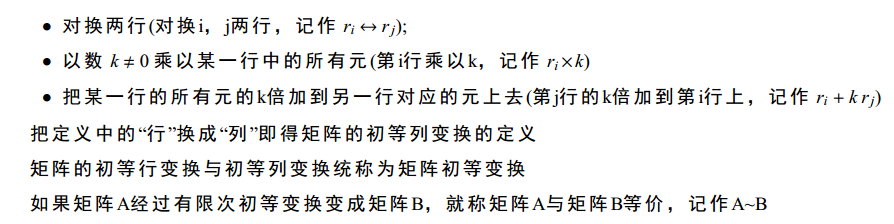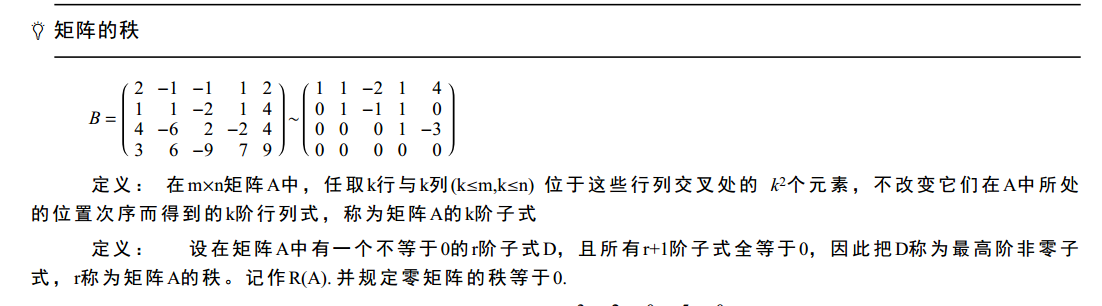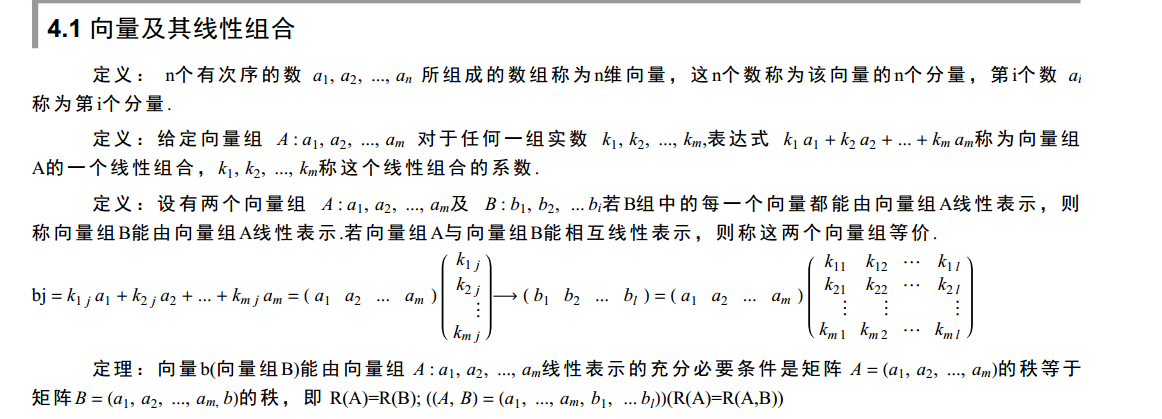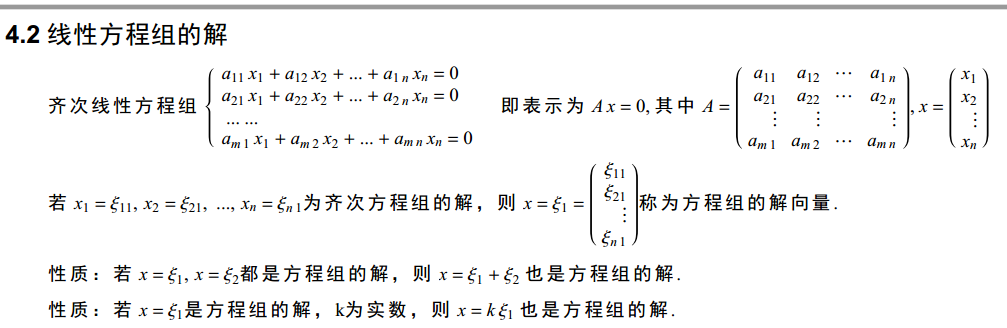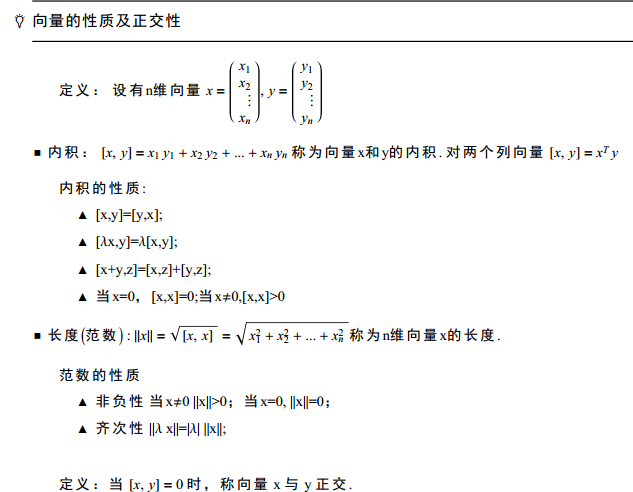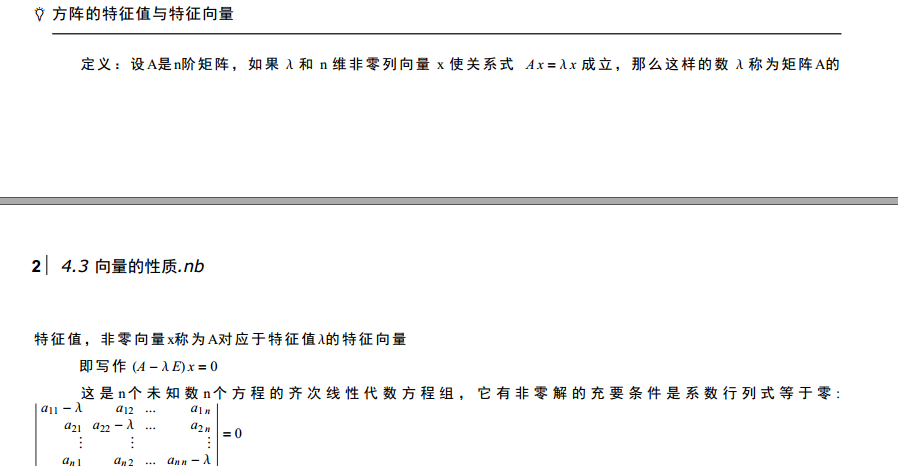a = np.array([[1,2,3],
[2,3,-5],
[4,7,1]])
b = np.array([[3,2,0,5,0],[3,-2,3,6,-1],[2,0,1,5,-3],[1,6,-4,-1,4]])
#计算秩
r1 = np.linalg.matrix_rank(a)
r2 = np.linalg.matrix_rank(b)
print(r1)
print(r2)
2
3
​
a = np.array([[3,-2,0,-1],[0,2,2,1],[1,-2,-3,-2],[0,1,2,1]])
print(np.linalg.inv(a))
print(np.linalg.det(a))
print(np.linalg.matrix_rank(a))
[[  1.   1.  -2.  -4.]
[  0.   1.   0.  -1.]
[ -1.  -1.   3.   6.]
[  2.   1.  -6. -10.]]
1.0000000000000004
4

​
a = np.array([[3,-2,0,-1],[0,2,2,1],[1,-2,-3,-2],[0,1,2,1]])
print(np.linalg.inv(a))
print(np.linalg.det(a))
print(np.linalg.matrix_rank(a))
[[  1.   1.  -2.  -4.]
[  0.   1.   0.  -1.]
[ -1.  -1.   3.   6.]
[  2.   1.  -6. -10.]]
1.0000000000000004
4

a = np.array([[-1,1,0],[-4,3,0],[1,0,2]])
print(np.linalg.eigvals(a))
print(np.linalg.eig(a))
[2. 1. 1.]
(array([2., 1., 1.]), array([[ 0.        ,  0.40824829,  0.40824829],
[ 0.        ,  0.81649658,  0.81649658],
[ 1.        , -0.40824829, -0.40824829]]))

a = np.array([[-2,1,1],[0,2,0],[-4,1,3]])
print(np.linalg.eigvals(a))
#对角化
np.diag(np.linalg.eigvals(a))

​

​
展开全文Python线性代数
• 一、相似矩阵 定义： 设A,BA,BA,B都是n阶矩阵，若有可逆矩阵PPP，使： 则称BBB是AAA的相似矩阵，或者说AAA和BBB相似。 1.1 线性变换： 首先从函数说起 1.1.1 线性函数： 函数直观的讲，就是把x轴上的点映射到曲线上...
一、线性变换
1.1 什么是线性变换
首先给出一个比较抽象的解释方式：
$对于一个变换A，找两个向量，如果这个变换满足可加性与齐次性：$
$A(\alpha+\beta)=A\alpha+A\beta$
$A(k\alpha)=k(A\alpha)$
$那么这个变换就是线性变换$
1.2 从函数角度理解
1.2.1 首先复习下函数
函数客观的讲就是把x轴上的点映射到曲线上，以下是一个正弦函数：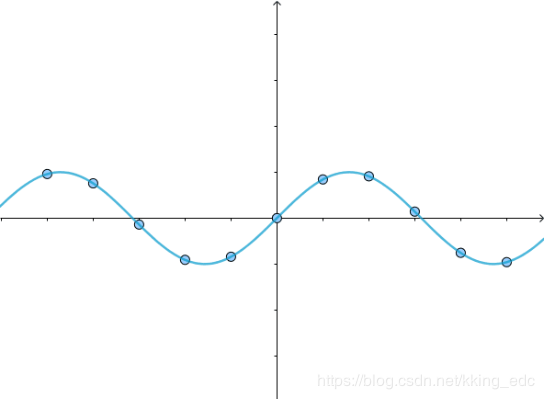1.2.2 线性函数
有的函数，比如y=x，是把x轴上的点映射到直线上，这种称之为线性函数：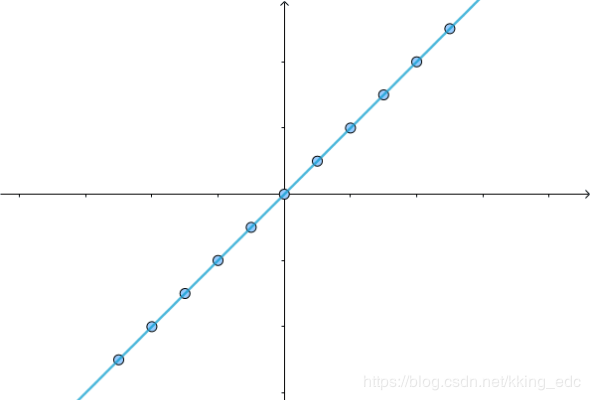1.3 从线性函数到线性变换
线性函数其实就是线性变换，为了看起来更像线性变换，这里换一种标记方法：
之前的y=x，可以认为是把$(a,0)$映射到了$(0,a)$点，这被称为线性变换T，记作：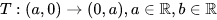矩阵的形式如下：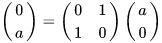这里将$(a,0)$替换为平面内所有的点$(a,b)$，我们就可以对整个平面做变换，该线性变换记作：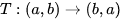写成矩阵的形式：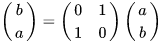我们记：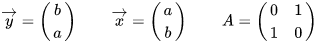这时可以得到一个更简便的记法（这种形式看起来更像线性方程$y=ax$）：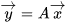我们已经假定$\overrightarrow{y},\overrightarrow{x}$指代了平面上所有的点，所以干脆可以更简化为：
线性变换通过矩阵A来表示
而y=x不过是这个A的一个特殊情况
1.4 矩阵A与基
刚才的结论其实是不完整的，还缺少了一个信息：
y=x是基于直角坐标系的，通过这个转换：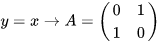得到的A也是基于直角坐标系的。
只是在线性变换中，我们不称之为直角坐标系，而是叫做标准正交基。
标准正交基是：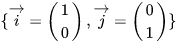它们张成的线性空间如下：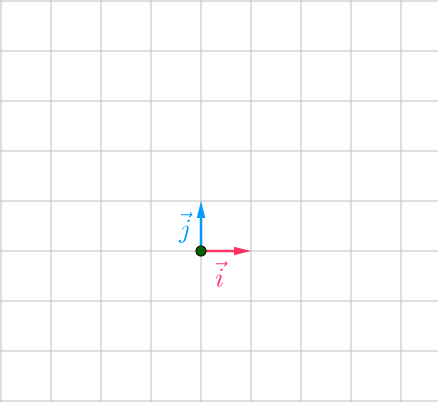这里，对前面的结论进行一个补充：
线性变换通过指定基下的矩阵A来表示
注意这个”指定基“，这说明基不一定固定为正交基，由此引出相似矩阵的概念。
二、相似矩阵
2.1 定义：
设$A,B$都是n阶矩阵，若有n阶可逆矩阵$P$，使：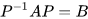则称$B$是$A$的相似矩阵，或者说$A$和$B$相似。
2.2 解释那怎么得到不同基下的矩阵呢? 这里看下具体的变换细节。
2.2.1 细节
首先看一个图，下面给出关于图的解释：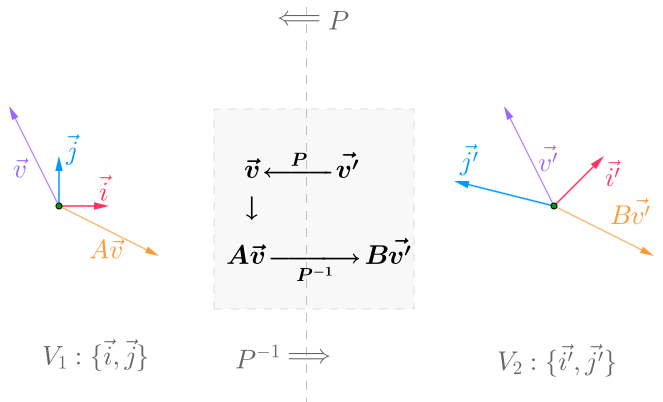有两个基：$V_1:\{\overrightarrow{i},\overrightarrow{j}\}$和$\{\overrightarrow{i'},\overrightarrow{j'}\}$
$V1\to V2$，可以通过$P^{-1}$转换
$V2\to V1$，可以通过$P$转换

整个转换的核心如下：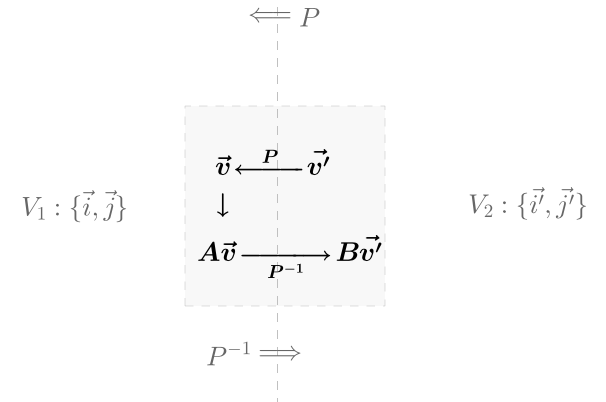对上面的图进行解释：

$\overrightarrow{v'}$是$V_2$的点
$\overrightarrow{v'}$通过$P$变为$V_1$下的点，即$P\overrightarrow{v'}$
在$V_1$下，通过矩阵$A$完成线性变换，即$AP\overrightarrow{v'}$
通过$P^{-1}$变回$V_2$下的点，即$P^{-1}AP\overrightarrow{v'}$

综上，我们可以有：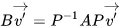我们可以认为：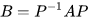那么B和A互为相似矩阵。
这里还有一个细节：$V_2\to V_1$的转换矩阵$P$是什么？
首先看空间中的一个点，假设为$m$点：这时我们知道，不管有没有基，这个点都是客观存在的，然后给出其在$\overrightarrow{i'},\overrightarrow{j'}$的坐标$\overrightarrow{v'}$：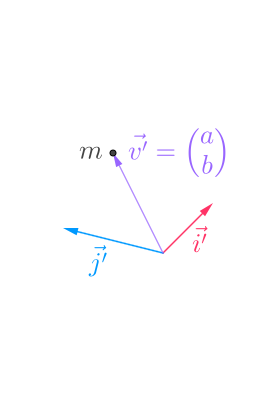为了表示$\overrightarrow{v'}$是$\overrightarrow{i'},\overrightarrow{j'}$下的坐标，我们写成这样：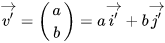如果我们知道了$\overrightarrow{i'},\overrightarrow{j'}$在$\overrightarrow{i},\overrightarrow{j}$下的坐标：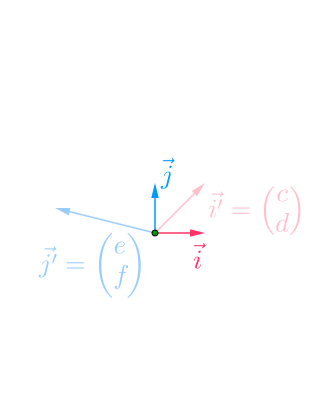那么有：
$\overrightarrow{v'}=a\overrightarrow{i'}+\overrightarrow{j'}=a(c\overrightarrow{i}+d\overrightarrow{j})+b(e\overrightarrow{i}+f\overrightarrow{j})$
此时，实际上m点的坐标，已经变到了$\overrightarrow{i},\overrightarrow{j}$下的$\overrightarrow{v}$：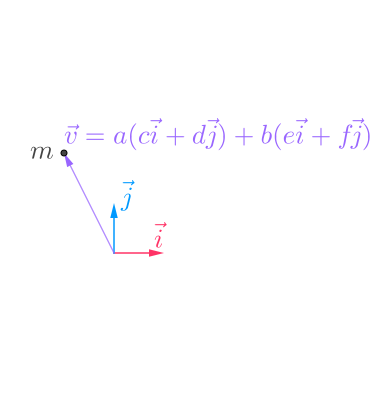继续推导：所以P其实就是：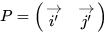这里的$\overrightarrow{i'},\overrightarrow{j'}$是在$\overrightarrow{i},\overrightarrow{j}$下的坐标。
2.2.2 对角矩阵
为什么我们需要相似矩阵呢？
比如A这个矩阵：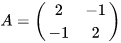可以这样分解：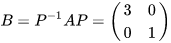其中：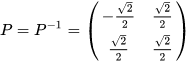B就是对角矩阵，看上去好看很多，相似变换其实就是坐标转换，转换到一个更方便计算的简单坐标系。
2.3 相似的性质：

反身性：$A\backsim A\quad(I^{-1}AI=A)$
对称性：$A\backsim B\rArr B\backsim A$
$(A\backsim B\rArr P^{-1}AP=B\rArr A=(P^{-1})^{-1}BP^{-1})$
传递性：$A\backsim B,B\backsim C,\rArr A\backsim C$
$P_1^{-1}AP_1=B,P_2^{-1}BP_2=C$
$\therefore P_2^{-1}P_1^{-1}AP_2P_1=C$
$\therefore (P_1P_2)^{-1}A(P_1P_2)=C$
相似矩阵的秩相同

三、对角矩阵
3.1 矩阵可对角化
如果矩阵$A$能与对角矩阵相似，则称$A$可对角化
例子：
设$A=\begin{bmatrix}1&1\\2&2\end{bmatrix},P=\begin{bmatrix}1&-1\\2&1\end{bmatrix}$ ，则有：
$P^{-1}AP=\begin{bmatrix}3&0\\0&0\end{bmatrix}$
即：$A\backsim\begin{bmatrix}3&0\\0&0\end{bmatrix}$
从而$A$可对角化
3.2 可对角化的条件
3.2.1 定理1：n阶矩阵A可对角化的充分必要条件是A有n个线性无关的特征向量
证明：
必要性：
如果A可对角化，则存在可逆矩阵P，使得：
$A=\begin{bmatrix}\lambda_1&0&0&\dots&0\\0&\lambda_2&0&\dots&0\\\vdots&\dots&\dots&\ddots&\vdots\\0&0&0&\dots&\lambda_n\end{bmatrix}$
将P按列分块得到$P=[X_1,X_2,...,X_n]$，从而有：
$AP=A[X_1,X_2,...,X_n]=P\begin{bmatrix}\lambda_1&0&\dots&0\\0&\lambda_2&\dots&0\\\vdots&\dots&\ddots&\vdots\\0&0&\dots&\lambda_n\end{bmatrix}=[X_1,X_2,...,X_n]\begin{bmatrix}\lambda_1&0&\dots&0\\0&\lambda_2&\dots&0\\\vdots&\dots&\ddots&\vdots\\0&0&\dots&\lambda_n\end{bmatrix}$
因此有：
$AX_i=\lambda_iX_i\quad(i=1,2,...,n)$，所以$X_i$是A的属于特征值$\lambda_i$的特征向量，又由P可逆，知$X_1,X_2,...,X_n$线性无关，故A有n个线性无关的特征向量。
3.2.2 定理2：矩阵A的属于不同特征值的特征向量是线性无关的
3.2.3 推论1：若n阶矩阵有n个互不相同的特征值$\lambda_1,\lambda_2,...,\lambda_n$，则A可对角化，且：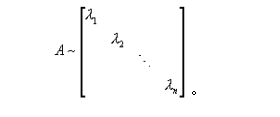3.2.4 定理三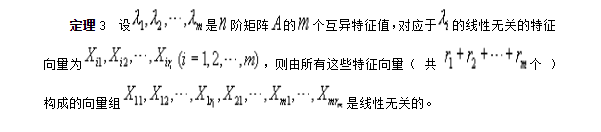3.3 对角矩阵的性质
3.3.1 对角矩阵的秩等于其对角线上非零元素的个数。
四、可逆矩阵
4.1 定义
设A为n阶方阵，若存在n阶方阵B，使得：
$AB=BA=I$
则称A为可逆矩阵，B为A的逆矩阵，记为$A^{-1}=B$
单位矩阵I：
$I^{-1}=I$
$(kI)^{-1}={1\over k}I,(k\ne0)$
对角矩阵：
D=$\begin{bmatrix}d_1&0&\dots&0\\0&d_2&\dots&0\\\vdots&\dots&\ddots&\vdots\\0&0&\dots&d_n\end{bmatrix},(d_1,d_2,...d_n\ne0);\quad D^{-1}=\begin{bmatrix}{1\over d_1}&0&\dots&0\\0&{1\over d_2}&\dots&0\\\vdots&\dots&\ddots&\vdots\\0&0&\dots&{1\over d_n}\end{bmatrix}$
4.2 定理
4.2.1 定理1：设A可逆，则它的逆是唯一的
证明：
设有B和C满足：AB=BA=I，AC=CA=I
则：B=BI=B(AC)=(BA)C=IC=C
4.2.2 定理2：设A为n阶矩阵，则下列命题等价：

A是可逆的
AX=0只有零解
$1\to2：设A是可逆的，且X是AX=0的解，则：$
$X=IX=(A^{-1}A)X=A^{-1}(AX)=A^{-1}0=0$
所以，AX=0只有零解
A与I行等价
$2\to3：A经过初等行变换到B（行阶梯矩阵）$
$BX=0只有零解，B的对角元均非零，否则B的最后一行的元全为零，则BX=0有非零解（矛盾）$
$则，B经初等行变换后得到的行最简化矩阵=I$
A可表为有限个初等矩阵的乘积
$3\to4：由3，可得A可经初等行变换得到I，所以存在初等矩阵E_1,E_2,...E_k，使得E_k,...E_1A=I$
$A=E_1^{-1}....E_k^{-1}I=E_1^{-1}...E_k^{-1}$

4.2.3 推论：设A为n阶矩阵，则AX=b有唯一解的充要条件是A可逆
证明：
充分性：
$AX=b有唯一解：X=A^{-1}b$
必要性：
$设AX=b有唯一解X，但A不可逆$
$A不可逆\rArr AX=0有非零解Z$
$令Y=X+Z$
$AY=A(X+Z)=AX+AZ=b+0=b$
$则Y为AX=b的解，矛盾$
所以可得A可逆
4.3 性质
设A，B皆为n阶可逆矩阵，数$\lambda\ne0$，则：

$A^{-1}$可逆，且$(A^{-1})^{-1}=A$
$\lambda A$可逆，且$(\lambda A)^{-1}={1\over\lambda}A^{-1}$
$AB$可逆，且$(AB)^{-1}=B^{-1}A^{-1}$
$(AB)(B^{-1}A^{-1})=A(BB^{-1})A^{-1}=AA^{-1}=I$
$A^T$可逆，且$(A^T)^{-1}=(A^{-1})^T$
$A^T(A^{-1})^T=(A^{-1}A)^T=I$
逆矩阵行列式和原矩阵行列式的关系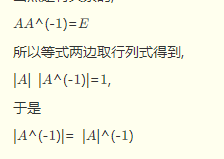https://wenku.baidu.com/view/84eda27b27284b73f24250ce.html?sxts=1591611918853
五、过渡矩阵
过渡矩阵是基与基之间的一个可逆线性变换，在一个空间V下可能存在不同的基。假设有两组基分别为A，B。由基A到基B可以表示为B=AP，过渡矩阵$P=A^{-1}B$，它表示的是基与基之间的关系。


展开全文• 已知矩阵A 相似矩阵B，借助初等变换的方法，可以构造性的获得演化矩阵P。即找到具体的可逆矩阵P，使B = P^(-1)AP，由B =P^(-1)AP，可得AP =PB，将P 的元素设为未知量，由矩阵的乘法及两矩阵相等可得一齐次线性方程...
已知矩阵A 相似于矩阵B，借助初等变换的方法，可以构造性的获得演化矩阵P。即找到具体的可逆矩阵P，使B = P^(-1)AP，由B =P^(-1)AP，可得AP =PB，将P 的元素设为未知量，由矩阵的乘法及两矩阵相等可得一齐次线性方程组，由方程组的一个非零解即可得到一个要求的演化矩阵P。
当然，这只是矩阵初等变换的一个小小的应用，它在线性代数中的更重要的应用主要体现于以下几点：求矩阵的秩，求向量组的极大无关组、秩，求解线性方程组，求多项式的最大公因式等。


展开全文• 本文主要介绍行列式的一些性质与应用，还有矩阵的一些运算 大概是《线性代数》的精简版外加一些自己的理解 行列式的定义： 令 ppp 为 1,2,...,n1,2,...,n1,2,...,n 的一个排列，排列中的逆序对个数为 ttt，那么...
• 第四节 二次型及其标准形 一．数学概念 1. 二次型  称含有n个变量 的二次齐次函数 为二次型。 ...1. 设可逆的线性变换x=Cy，将f化成标准形，即  其实质将对称矩阵A化成对角阵L。 2. 任给可线性代数
• 初等变换矩阵、向量组的秩 零矩阵的判定方法 向量空间、正交矩阵与线性变换 方阵的迹及其性质 矩阵的特征值 相似变换与相似对角化 复数的运算法则、复矩阵的共轭与共轭转置 复数的运算法则 ...线性代数 机器学习的数学基础
• 可逆矩阵（定义、充要条件、与初等矩阵）、分块矩阵相似对角化、正交矩阵（定义、充要条件及性质）
• 给出了矩阵相似的两个充分必要条件，讨论了相似问题中的可逆矩阵初等变换求解方法．只要对两个矩阵的特征矩阵进行初等变换化简，就可以判断是否相似，并在相似时通过简单计算求得相应的可逆矩阵
• 相似矩阵要点总结】其实整个相似矩阵的重点就在以下四张图里，算法涉及到三阶带参行列式的求解、初等变换求解方程组、和施密特正交化，只是这一章我们新学了很多概念和知识点，需要在不同的情境下去使用这个算法...c++求矩阵的秩 两矩阵相乘的秩的性质
• ## 相似矩阵、矩阵的相似对角化

万次阅读 多人点赞 2016-10-19 19:12:47
相似矩阵的定义A,B都是n阶矩阵。若存在可逆矩阵P，使得P−1AP=BP^{-1}AP=B,则称A相似于B，记作A∼BA \sim B。 特殊的，如果A∼Λ,Λ是对角矩阵A \sim \Lambda, \Lambda 是对角矩阵, 则称A可以相似对角化。Λ\Lambda...线性代数
• 文章目录初等因子的概念初等因子与不变因子的求法参考 初等因子的概念 定义7\large\color{magenta}{\boxed{\color{brown}{定义7} }}定义7​ 把矩阵 A(A(A( 或线性变换 A)A)A) 的每个次数大于零的不变因子分解成互不...
•算法 线性代数 矩阵运算 矩阵论
• 在做矩阵的逆运算（也就是出除运算）时，分母的左右取决余原乘式左右； 右除式A/B，相当于A*inv(B)即A右乘B的逆矩阵； 左除式A\B，相当于inv（A）*B即A的逆矩阵左乘B ...
• 线性代数张宇9讲 第八讲 相似矩阵与相似对角化 易错题和难题记录线性代数
• 两个相似矩阵有许多相同的性质： 两者的秩相等。两者的行列式相等。两者的迹数相等。两者拥有同样的特征值，尽管相应的特征向量一般不同。两者拥有同样的特征多项式。两者拥有同样的初等因子。
• 如何用MATLAB矩阵如果英文好呢，自己看目录不好还是先看中文的教材，对matlab的框架和功能有了一定的了解后，自己也就看的懂帮助里面的内容了，以后不懂再自己查帮助矩阵一般有2种方法：1、伴随矩阵法。...
• 如果矩阵A经过有限次初等变换变成矩阵B，就称矩阵A与B等价。 性质 反身性：A~A 对称性：若A~B，则B~A 传递性：若A~B,B~C,则A~C 推论： 有两个m×n阶矩阵A和B，如果这两个矩阵满足B=QAP（P是n×n阶可逆...线性代数
• 设A是n阶矩阵，α是n维非零列向量，满足：Aα=λα，(1)Aα=λα，\tag1Aα=λα，(1)则称λ是A的一个特征值。非零列向量是A的属于λ的一个特征空间。 式（1）可以写成 (λEn−A)X=0(λE_n-A)X=0 (λEn​−A)X=0 它...线性代数
• 矩阵A（或线性变换）的每个次数大于零的不变因子分解成互不相同的首项为1的一次因式方幂的乘积，所有这些一次因式方幂（相同的必须按出现的次数计算）称为矩阵A（或线性变换）的初等因子。 设一个n级矩阵的全部...
• 矩阵相似的定义和矩阵等价的定义可以参考线性代数和矩阵论相关的...对于矩阵等价问题来说，PAQ = B，表示对A进行一系列的初等变换，将A变成B矩阵，同样的，对该式做变换，将左侧的P乘到右侧，变成： A*Q = P^(-1)*B，
• 做论文需要用相似矩阵评估一个聚类模型。我不需要知道其数学原理，只要知道这东西是干什么用的。有人能告诉我么？ ＝＝＝＝＝＝＝＝＝＝＝＝＝＝＝＝＝＝＝＝＝ 补充一下，我知道什么是相似矩阵，也知道其各种数学...
• ## 矩阵求秩

万次阅读 多人点赞 2019-05-13 17:20:08
矩阵的秩怎么计算，这个问题一下子我居然不知道怎么下手。。虽然本科的时候学过线性代数，但是好久不用，很多...矩阵的秩是方阵经过初等变换或者列变换后的行秩或列秩 矩阵的秩是线性代数中的一个概念。在线性代...
• 等距变换(Isometries) [x′y′1]=[σcos⁡θ−sin⁡θx0σsin⁡θcos⁡θy0001][xy1]\left[\begin{matrix}x'\\y'\\1\\\end{matrix}\right]= \left[\begin{matrix}\sigma\cos\theta&-\sin\theta&x_0\\\sigma\...计算机视觉 线性代数 3d
• 线代中其他的一些遗留小问题，后续可能会更新。 1. 初等变换不改变什么？（初等变换同理） 2. 特征方程的简便设法。 3. 典型错题
• 如果矩阵A经过有限次初等变换变成矩阵B，就称矩阵A与B等价。 性质 反身性：A~A 对称性：若AB，则BA 传递性：若AB,BC,则A~C 推论： 有两个m×n阶矩阵A和B，如果这两个矩阵满足B=QAP（P是n×n阶可逆矩阵，Q是m×m阶...
• 分为如下六个部分： - 线性变换及其运算 - 线性变换矩阵表示 - 特征值与特征向量 - 对角矩阵 - 不变子空间 - Jordan 标准形
• estimateRigidTransform()：计算多个二维点对或者图像之间的最优仿射变换矩阵 （2行x3列），H可以是部分自由度，比如各向一致的切变。 getAffineTransform()：计算3个二维点对之间的仿射变换矩阵H（2行x3列），...
• 怎么矩阵对应的基呢？ 对矩阵做初等变换，化为上三角形 或 对角型， 主对角元素不为0的列即为该矩阵的一组基。 A = 这个矩阵对应的一个基 为 , , ...可求得，A矩阵的一个相似矩阵 B = , ...
• 利用线性变换（映射）的值域空间、核子空间和不变子空间进行映射性质的求解以及表示矩阵的简化。线性代数 笔记 数学...